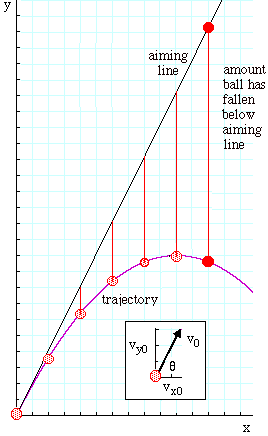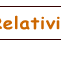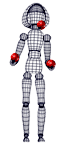# The monkey and the hunter

• How far does a projectile 'fall away' from the line along which it is aimed?

• What happens if you aim at a target, and if the target begins to fall just as the projectile is launched?

The Monkey and the Hunter is a physics question so old that it has a heritage listing. As far as we know, it is purely imaginary. A monkey hangs from a tree. A hunter aims a rifle at him and fires. At the instant that the gun fires, the monkey lets go of the branch and begins to fall, thinking that he will thus fall below the trajectory of the bullet. (Monkeys don't study physics.) What happens?

 Before doing the mathematics, let's look at the situation. The monkey falls and accelerates downwards at g. The bullet starts off travelling along the aiming line, but it is also accelerated downwards at g. So we can imagine its motion as 'falling below the aiming line'. At equal times, it will fall below this line by an amount equal to the distance fallen by the monkey. At the time when both have the same horizontal position, they will both have fallen the same distance. This is not good news for the monkey. The aiming line or sighting line is the path taken by light, which is not affected by gravity (or at least not measurably affected by the Earth's gravity) and so is a straight line - the black line in this graph. If the projectile is fired along this line, its initial velocity, v0, is along that line. If air resistance may be neglected, then there are no horizontal forces and so the horizontal component of velocity, vx, is constant. The vertical component, on the other hand, is steadily decreased by the acceleration due to gravity. The resulting trajectory is shown at right. The sequential positions of the bullet shown at right are equally spaced, 2 units apart. Because the horizontal component of the velocity vx is constant, then these represent equally spaced times and the horizontal position x is just vxt. The vertical lines between the aiming line and the trajectory show how far the bullet has fallen below the aiming line. The vertical component of velocity vy has an initial value vy0, but decreases due to gravity: vy = vy0 - gt. Integrating this with respect to time gives us the vertical position of the projectile: y = y0 + vy0t - gt2/2. We can think of the aiming line as the path of an imaginary projectile that was not affected by gravity. This would be: yaim = y0 + vy0t . The amount by which the projectile has fallen below the aiming line is Δy = yaim - y = gt2/2. Δy is the height of the vertical red lines in the figure, which increase in the ratio 0, 1, 4, 9, . . . n2. Now if you look at the algebra above and put v0 = 0, you will see that gt2/2 is the distance fallen in time t by an obect starting with zero vertical velocity. So the monkey falls as far below the aiming line as the bullet does. No monkeys were hurt in the making of this clipMore formally, we could use the standard equations for motion in the x and y direction. Let the bullet start from (x0,y0) with velocity components vx0 and vy0 at t = 0. If we neglect air resistance, there is no acceleration in the x direction, so
x = vx0t    so       t = x/vx0.
In the y direction, the acceleration is -g, so
y = vy0t - ½gt2,
which is the equation for a parabola in y(t): a parabola on a displacement-time graph. Combining the two equations above to eliminate t gives
y = x(vy0/vx0) - (½g/vx02)x2,
which is the equation for a parabola in space, in other words the trajectory of the ball. Now v0, the initial velocity, is parallel to the aiming line, and is the tangent to the trajectory at t = 0, as shown in the diagram. So we can substitute tan θ = (vy0/vx0) and get
y = (tan θ)x - (½g/vx02)x2.
The aiming line is just
yaim = tan θ.x
which we could get from the preceding equation by setting g = 0, because light is not affected by gravity (or at least not very much by the Earth's relatively weak field). So the vertical lines in the figure are
Δy = yaim - y = ½(g/vx02)x2
and, using x = vx0t again, we have

Δy = yaim - y = ½gt2,

which expresses in an equation what we wrote above: the monkey falls as far below the aiming line as the bullet does.

 Thanks to Steven Preece, George Hatsidimitris, Gary Keenan and Tamara Reztsova for high speed videos, flash animations, aiming and monkey wrangling. Joe Wolfe | J.Wolfe@unsw.edu.au | School of Physics, University of New South Wales, Sydney, Australia.

As of June 2005, relativity was 100 years old. Our contribution is Einstein Light: relativity in brief... or in detail. It explains the key ideas in a short multimedia presentation, which is supported by links to broader and deeper explanations.This work is licensed under a Creative Commons License.